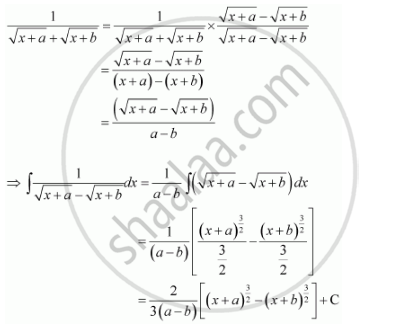Share

# Integrate the Functions 1/(Sqrt(X+A) + Sqrt(X+B)) - CBSE (Science) Class 12 - Mathematics

ConceptIntegration as an Inverse Process of Differentiation

#### Question

Integrate the functions 1/(sqrt(x+a) + sqrt(x+b))

#### SolutionIs there an error in this question or solution?

#### Video TutorialsVIEW ALL 

Solution Integrate the Functions 1/(Sqrt(X+A) + Sqrt(X+B)) Concept: Integration as an Inverse Process of Differentiation.
S